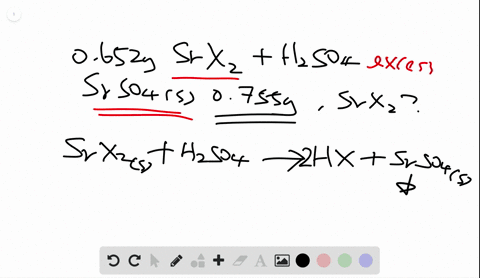🎉 The Study-to-Win Winning Ticket number has been announced! Go to your Tickets dashboard to see if you won! 🎉View Winning Ticket### A 0.652 -g sample of a pure strontium halide reac…

06:15University of Maine
Problem 108

# During studies of the reaction in Sample Problem 3.20 $$2 \mathrm{N}_{2} \mathrm{H}_{4}(l)+\mathrm{N}_{2} \mathrm{O}_{4}(l) \longrightarrow 3 \mathrm{N}_{2}(g)+4 \mathrm{H}_{2} \mathrm{O}(g)$$a chemical engineer measured a less-than-expected yield of $\mathrm{N}_{2}$ and discovered that the following side reaction occurs:$$\mathrm{N}_{2} \mathrm{H}_{4}(l)+2 \mathrm{N}_{2} \mathrm{O}_{4}(l) \longrightarrow 6 \mathrm{NO}(g)+2 \mathrm{H}_{2} \mathrm{O}(g)$$In one experiment, 10.0 g of NO formed when 100.0 g of eachreactant was used. What is the highest percent yield of $\mathrm{N}_{2}$ that canbe expected?

## Discussion

You must be signed in to discuss.

## Video Transcript

given an equation in which you're given amounts of reactive issue could predict the amount of product that will form. This is called the Theoretical Yield. So in this case, we have 100 grams of each reacted and the product that we're looking for is end to because we have to amounts of reactant. One of them is the limiting re agent, so Onley one will determine how much product is formed. So the way to find this is too. First, convert each quantity two moles when we do this using the Moeller math. And then we can change from moules of the reactant two moles of the product that we do this using the mole ratio from the balanced equation. So we calculate this for both reactant and whichever smaller is our theoretical yield. To find the molar mass of each substance, we use the periodic table and we add the atomic masses of each substance. Time to sub script. So for into H four, it'll be two times 14.7 plus four times 1.8 or the more mass his 32.0 for six grams. Similarly, the molar mass for end to 04 is found by multiplying two times 14.7 plus four times oxygen, which is 15.994 Just 92 went 01 grams. We also need the molar mass of our product and to which is two times 14 with 007 We're 28.14 grams. If I have 100 grams of end to four h four, my first step is to change two moles dividing by molar Mass. And then I can change two moles because I know that there are three moles of nitrogen for every two moles and two h four. And so this amount would produce 4.68 moles and to similarly 100 grams and 204 changing two moles by dividing by the molar mass multiplying by the mole ratio, which is three moles and two for every one mole and two of four I find this produces 3.26 morals. Since this is the smaller quantity, that's the theoretical yield. We can change it to grams by multiplying by the molar mass of nitrogen. So my theoretically yield is 91.34 grams to find out how much is actually produced. We have to consider this side reaction. This will tell us how much nitrogen is taken away from the reaction in this case. I know that I'm producing 10 grams of N O, and I want to find how many grams of nitrogen that's equivalent to. So the first step is to change that two moles using the molar Mass by adding up nitrogen and oxygen. That's 30 wait 006 grams, and then I can find a relationship between my moles of nitrogen monoxide and a compound that's found in the previous equation. So in this case, I'll use my nature Die nitrogen tetroxide. I can then go back to the first reaction and see that that relates to the mole ratio of nitrogen. There are three moles of end to for everyone more of end to 04 and then finally, I can convert that two grams of nitrogen, and so if 10 grams of nitrogen monoxide is reacted, it should use up 9.34 grams of nitrogen. So the actual yield is how much I should have produced or 91.34 minus how much was taken away by the side reaction. We're 82 grams. I can then find my percent you by dividing my actual by my theoretical times, 100 commit 89.8% yield.